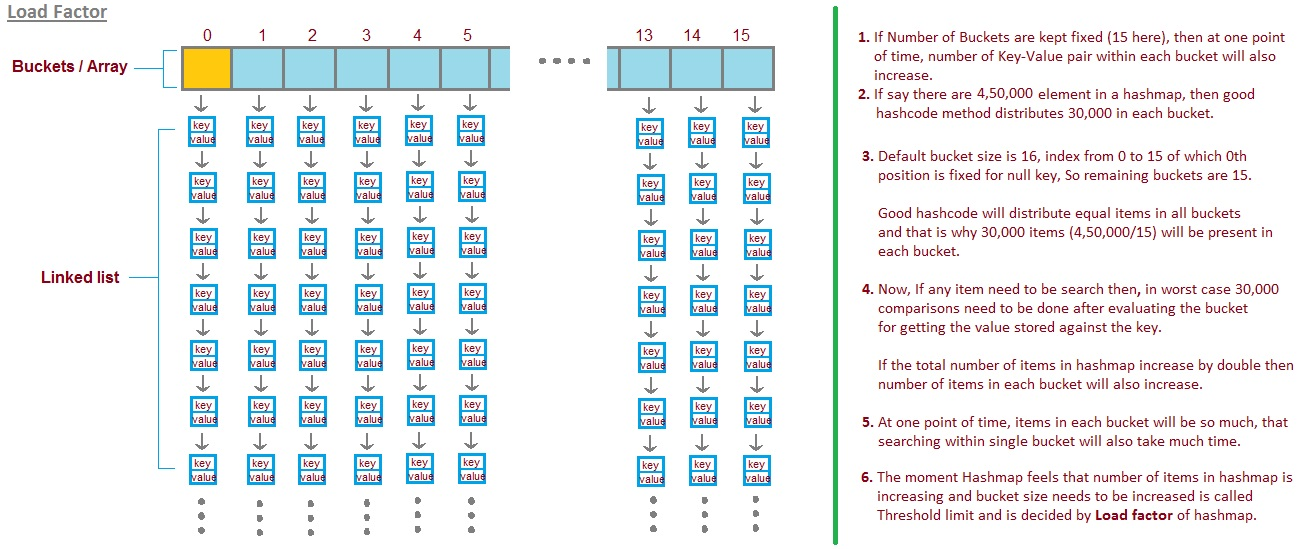Date Location, Data Structures and Algorithms final projectJava
موضوع‌ها
۰
فورک‌ها
۰
ستاره‌ها
۷
تاریخ ایجاد
۲۱ دی ۱۴۰۰
آخرین بروزرسانی
۷ ماه قبل

# DS-Final-Project

Date Location, Data Structures and Algorithms final project implemented in Java

# Description

Date Location is the final project of the Data Structures and Algorithms course in the fall 2021 semester at AUT.
In this project, we had to implement a system which would get a modeled graph of city junctions besides the nodes of candidate people who wanted to go to the best cafe (by best, we mean the cafe which the sum of differences between the distance to be traveled by candidates is minimized).

To solve this problem, we had to implement three phases in which we solve some parts of the problem.

## Phase 0

In this phase first, we need to input a modeled graph and store it in our system. To do this, we use a hash map to map each node label to its corresponding node. Then by using a dynamic list (specifically ArrayList in Java), we store adjacent edges to each node.
After that, we needed to run DFS algorithm to achieve DFS sequence.

## Phase 1

After saving the graph and accomplishing DFS algorithm, we had to iterate over all nodes in the order of DFS sequence and for each node, calculate the score of the corresponding node, based on a given formula. To calculate this score, we had to have the distance between candidate nodes and the current node. In order to achieve this, we ran a Dijkstra algorithm to compute these distances.

After calculating the score for each node, we chose the one with the highest score and report it to the user.

## Phase 2

In the final phase, we had to optimize our previous algorithm by some preprocessing on nodes' distances. To make this optimization practical, we had to implement a custom hash table to store distances. In order to make a hash table, first of all, we need to have a basic hash function that has to be distributed. After that, we implemented an array with dynamic size in which every position is a separate linked list (we call this position a single bucket), so we can handle collisions by a linked list. Better understanding can be achieved using the image below: (image source)By implementing bucket class and hash function, the only thing left is implementing dynamic array size. In order to obtain this data structure, we defined two factors called shrinking coefficient and extending coefficient (also called load factor), which will specify the size of the array in which we will shrink/extend it.

After completing these implementations, we just had to run Dijkstra algorithm over all nodes and store distances in the custom hash tables.